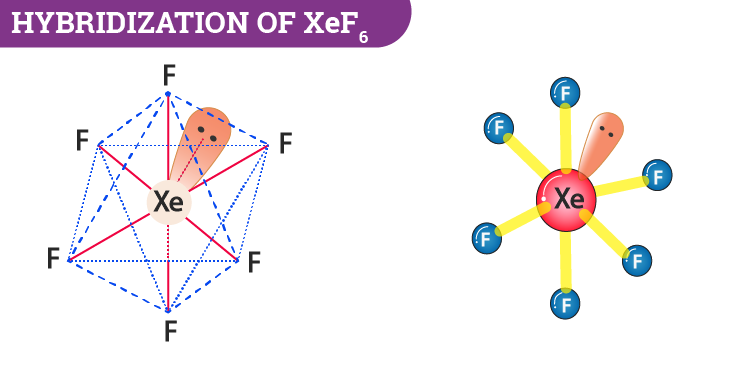# Hybridization of XeF6 (Xenon Hexafluoride)

During hybridization of XeF6, the molecule is sp3d3 hybridized. Here we learn how to determine the type of hybridization and understand the formation of a certain molecular geometry in xenon hexafluoride along with the bond angles.

 Name of the Molecule Xenon Hexafluoride Molecular Formula XeF6 Hybridization Type sp3d3 Bond Angle 90o and 72o Geometry Distorted Octahedral

## What is the Hybridization of Xenon Hexafluoride?

We can easily determine the hybridization of xenon hexafluoride by using the common formula which is;

 Hybridization=1/2[V+M-C+A]

Here,

v = number of valence electrons,

m = monovalent

c = positive charge

a = negative charge

Let us put the values according to the formula.

Hybridization = ½ [8+6-0+0]

= ½ 

= 7

The hybridization number is equal to 7. Now we can say that hybridization is sp3d3.

Alternatively, we can also determine the hybridization of I3- by knowing the number of bond pairs and lone pairs. During the formation of XeF6, xenon has 8 electrons in its valance shell and it forms six bonds with the fluorine atoms. Further, the molecules will have one lone pair and 6 bond pairs. Now if we take the steric number then it will be 7. This can be interpreted as sp3d3 hybridization.### Important Points To Remember

• XeF6 has seven electron pairs. It consists of 6 bond pairs and one lone pair.
• Xenon has 8 electrons in its valance shell and it forms six bonds with the fluorine atoms.
• When the fluorides of xenon have formed the electrons in the valence shell of xenon get unpaired and are promoted to vacant 5d orbitals.

## XeF6 Molecular Geometry And Bond Angles

After hybridization XeF6 molecular geometry will be distorted octahedral or square bipyramidal. What happens here is that the fluorine atoms are placed in the vertices of the octahedron while the lone pairs move in the space to avoid or reduce the repulsion.